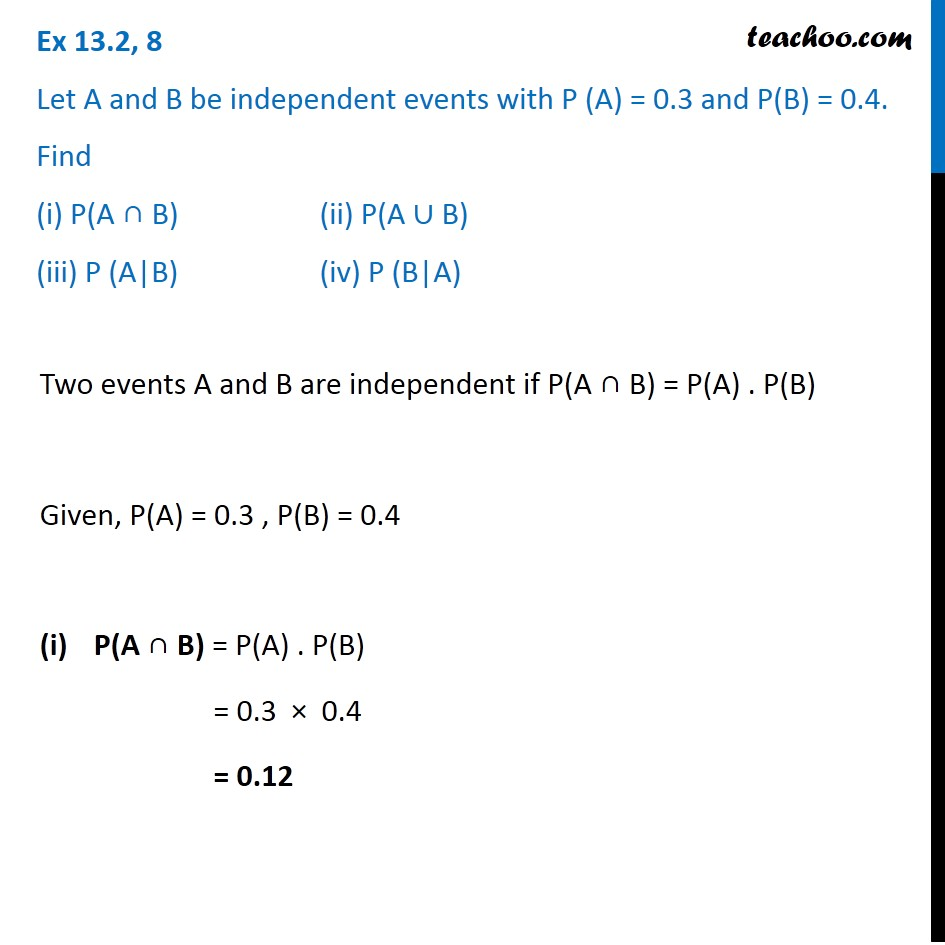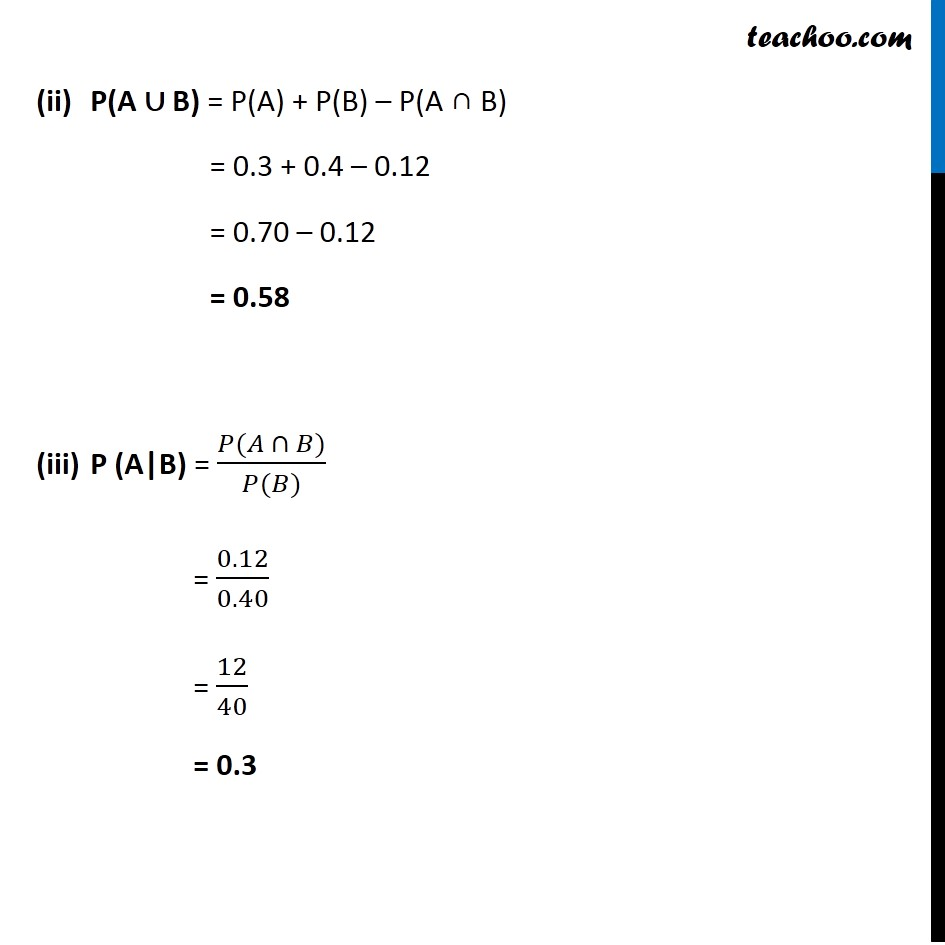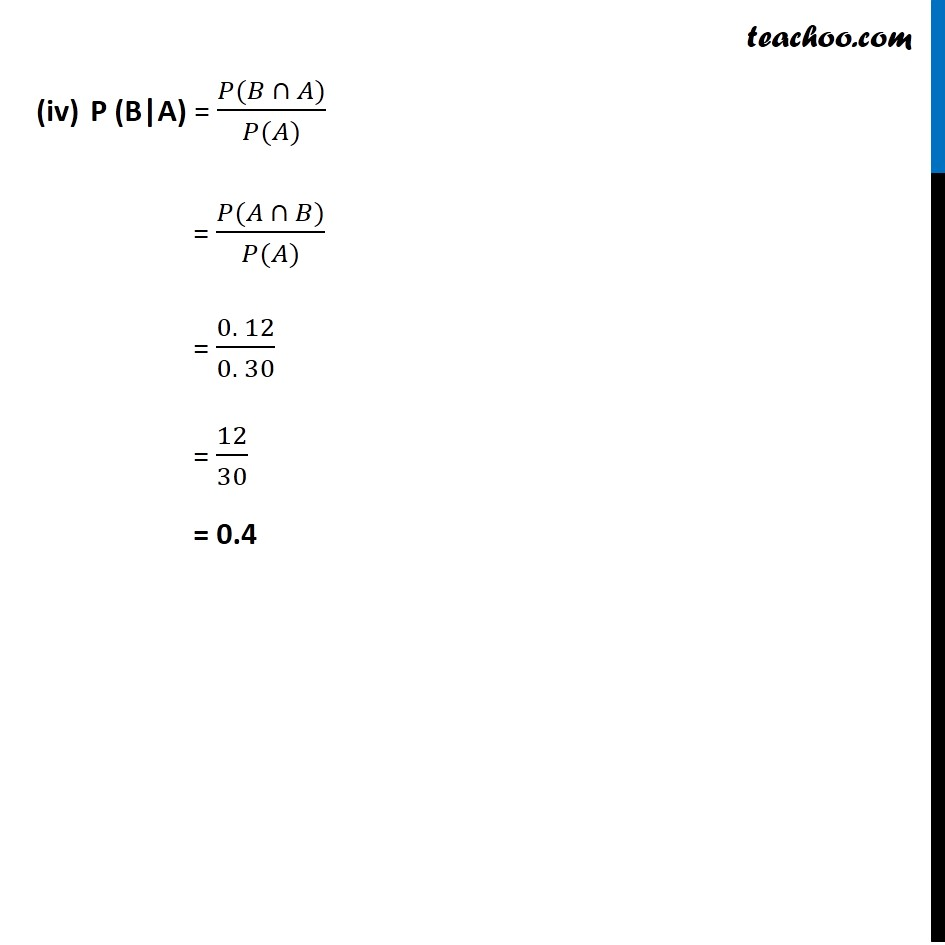Ex 13.2

Chapter 13 Class 12 Probability
Serial order wiseGet live Maths 1-on-1 Classs - Class 6 to 12

### Transcript

Ex 13.2, 8 Let A and B be independent events with P (A) = 0.3 and P(B) = 0.4. Find (i) P(A ∩ B) (ii) P(A ∪ B) (iii) P (A|B) (iv) P (B|A)Two events A and B are independent if P(A ∩ B) = P(A) . P(B) Given, P(A) = 0.3 , P(B) = 0.4 P(A ∩ B) = P(A) . P(B) = 0.3 × 0.4 = 0.12 P(A ∪ B) = P(A) + P(B) – P(A ∩ B) = 0.3 + 0.4 – 0.12 = 0.70 – 0.12 = 0.58 P (A|B) = (𝑃(𝐴 ∩ 𝐵))/(𝑃(𝐵)) = 0.12/0.40 = 12/40 = 0.3 P (B|A) = (𝑃(𝐵 ∩ 𝐴))/(𝑃(𝐴)) = (𝑃(𝐴 ∩ 𝐵))/(𝑃(𝐴)) = (0. 12)/(0. 30) = 12/30 = 0.4